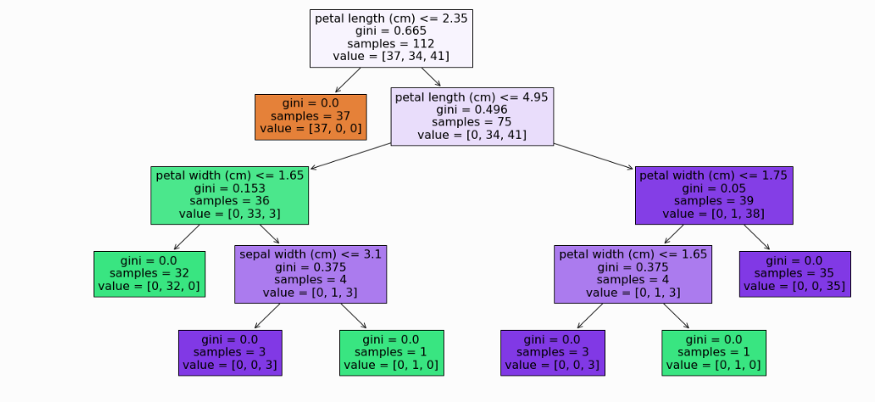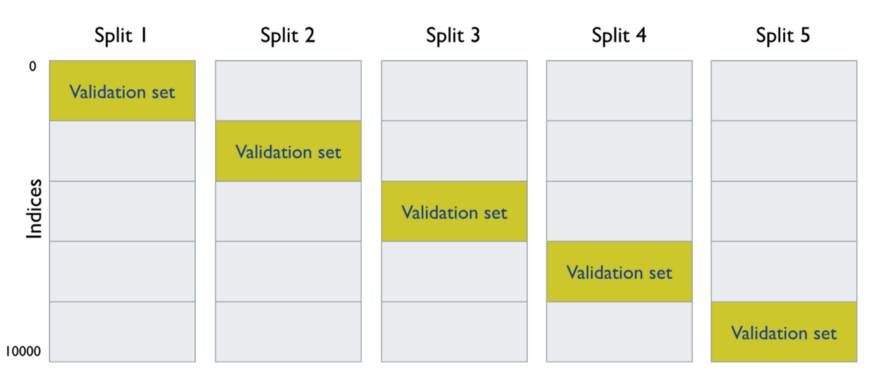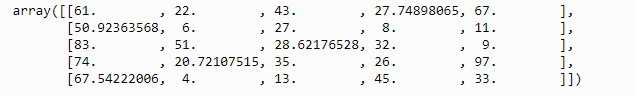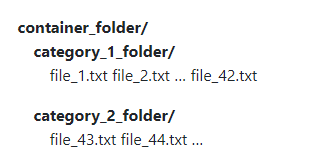14 Lesser-Known Features of Scikit Learn Library
Technical · 14 Jan 2021, 10:14 · 14 mins read ·
1

Do you use Scikit learn? Here are Davis David's favourite often unknown and underrated functionalities in scikit learn library.

Scikit learn remains one of the most popular open-source and machine learning library in the world of Artificial Intelligence. The Scikit-learn library contains a lot of efficient tools for machine learning and statistical modeling including classification, regression, clustering, and dimensionality reduction.

Scikit-learn is largely written in Python programming language and some core algorithms are written in Cython to improve its performance. It also integrates well with many other Python libraries, such as Matplotlib and Plotly for plotting, NumPy for array vectorization, Pandas dataframes, Scipy, and many more.Scikit-learn comes loaded with a lot of features. Here are a few of them

• Datasets
• Feature extraction
• Feature selection
• Parameter Tuning
• Clustering
• Cross-Validation
• Supervised Models
• Unsupervised Models
• Dimensionality Reduction
• Ensemble methods

Scikit learn has more than 1770 contributors and 41.2k stars on its GitHub repository, this means many data scientists, machine learning engineers and researchers rely on this library for machine learning projects. I personally love using the Scikit-learn library because it offers a ton of flexibility and its documentation is easy to understand with a lot of examples. In this article, I’m happy to share with you lesser-known impressive features in the Scikit-learn library that you did not know existed.

### 1. Clone Estimator

If you want to duplicate an estimator and use it on another dataset clone function can help you do that. A clone function helps you to constructs a new estimator with the same parameters.

“Clone does a deep copy of the model in an estimator without actually copying the attached data. It yields a new estimator with the same parameters that have not been fit on any data.”- scikit learn documentation

Example:

We start by creating a classification dataset and estimators.

`from sklearn.linear_model import LogisticRegression`
`from sklearn.datasets import make_classification`
`# create dataset``
`X1, y1 = make_classification(n_classes=2, n_features=5, random_state=1)`
`# create estimators `
`logistic_classifier_1 = LogisticRegression()`

Now we will use the clone function from sklearn.base to duplicate the logistic_classifier_1 model.

`from sklearn.base import clone`
`# duplicae the first classifier with clone function `
`logistic_classifier_2 = clone(logistic_classifier_1)`
`logistic_classifier_2`

The output of the new estimator named logistic_classifier-2 cloned from logistic_classifier_1 is as follows.

`LogisticRegression(C=1.0, class_weight=None, dual=False, fit_intercept=True,`
`                   intercept_scaling=1, l1_ratio=None, max_iter=100,`
`                   multi_class='auto', n_jobs=None, penalty='l2',`
`                   random_state=None, solver='lbfgs', tol=0.0001, verbose=0,`
`                   warm_start=False)`

### 2. Identify estimators as classifiers or regressors

You can identify a model instance if it solves a classification or regression task in the Scikit-learn library with two simple functions is_classifier and is_regressor. is_classifier function returns True if the given estimator is a classifier and is_regressor returns True if the given estimator is a regressor.

Example:

Start by creating two estimators, the first one as regression and the second one as classification.

`from sklearn.linear_model import LinearRegression`
`from sklearn.ensemble import RandomForestClassifier`
`# create estimators `
`model_1 = LinearRegression()`
`model_2 = RandomForestClassifier()`

Let check if the first model is regression.

`# check if it is regressor`
`from sklearn.base import is_regressor`
`is_regressor(model_1)`

The output is True.

Now let check if the second model is classification.

`# check if it is classifier `
`from sklearn.base import is_classifier`
`is_classifier(model_2)`

The output is True

### 3.Select columns with make_column_selector

Use make_column_selector with make_column_transformer to apply different preprocessing to different columns according to their data types (integers, categories) or column names.

Example:

In this example, we use make_column-_selector to select all object features in the dataset and transform them by using the OneHotEncoder method.

`import numpy as np `
`import pandas as pd`
`from sklearn.preprocessing import StandardScaler, OneHotEncoder`
`from sklearn.compose import make_column_transformer`
`from sklearn.compose import make_column_selector`
`# create a dataframe with different data types`
`data = pd.DataFrame(`
` {“gender”: [“male”, “female”, “female”, “male”],`
` “age”: [23, 5, 11, 8]}`
`)`
`# create a column transformer with make_column_selector`
`ct = make_column_transformer(`
` (StandardScaler(), make_column_selector(dtype_include=np.number)), # ages`
` (OneHotEncoder(), make_column_selector(dtype_include=object)), # genders`
`)`
`transformed_data = ct.fit_transform(data)`
`transformed_data`

The output of the transformed data is:

`array([[ 1.6464639 ,  0.        ,  1.        ],`
`       [-0.98787834,  1.        ,  0.        ],`
`       [-0.10976426,  1.        ,  0.        ],`
`       [-0.5488213 ,  0.        ,  1.        ]])`

### 4. Plotting the decision tree

You can visualize a decision tree model with plot_tree function. The plot function allows you to add features name with a parameter called feature_names.

Example:

We start by creating a classification model for the Iris dataset by using the Decision tree algorithm and then plot the decision tree.

`# import libraries`
`import matplotlib.pyplot as plt `
`from sklearn.model_selection import train_test_split`
`from sklearn.metrics import plot_confusion_matrix`
`from sklearn.tree import DecisionTreeClassifier, plot_tree, export_text `
`from sklearn.datasets import load_iris`
`#load data `
`iris = load_iris()`
`# create our instances`
`model = DecisionTreeClassifier()`
`# train test split`
`X_train, X_test, y_train, y_test = train_test_split(iris.data, iris.target, random_state = 0)`
`# fit and predict`
`model.fit(X_train, y_train)`
`# plot the tree`
`plt.figure(figsize = (20, 10))`
`plot_tree(model,feature_names=iris.feature_names, filled = True) `
`plt.show()`

We passed the model and feature names in the plot_tree function to create a decision tree plot.### 5. Fetch dataset from Openml

Openml is an online platform that aims at improving Open Machine Learning by providing an open, collaborative, frictionless, automated machine learning environment.

You can fetch the dataset from the Openml platform by using fetch_openml function from Scikit-learn.

Example:

Fetch bank-marketing dataset by using the name.

`from sklearn.datasets import fetch_openml`
`#fetch by using data name`
`bank_marketing = fetch_openml(name=”bank-marketing”)`
`# seperate independent variables and target variable `
`x = bank_marketing.data `
`y = bank_marketing.target`

Access sample of the fetched dataset.

`x[:2]`

The output is

`array([[ 5.800e+01,  4.000e+00,  1.000e+00,  2.000e+00,  0.000e+00,`
`         2.143e+03,  1.000e+00,  0.000e+00,  2.000e+00,  5.000e+00,`
`         8.000e+00,  2.610e+02,  1.000e+00, -1.000e+00,  0.000e+00,`
`         3.000e+00],`
`       [ 4.400e+01,  9.000e+00,  2.000e+00,  1.000e+00,  0.000e+00,`
`         2.900e+01,  1.000e+00,  0.000e+00,  2.000e+00,  5.000e+00,`
`         8.000e+00,  1.510e+02,  1.000e+00, -1.000e+00,  0.000e+00,`
`         3.000e+00]])`

You can also fetch data by using the specified ID.

`# fetch by using id from this link https://www.openml.org/d/1461`
`bank_marketing = fetch_openml(data_id=1461)`
`# seperate independent variables and target variable `
`x = bank_marketing.data `
`y = bank_marketing.target`

In the example above we fetch data with an ID of 1461.

### 6. Learning curve

Learning curve function from Scikit-learn let you determine the cross-validated training and test scores for different training set sizes.

“A cross-validation generator splits the whole dataset k times in training and test data. Subsets of the training set with varying sizes will be used to train the estimator and a score for each training subset size and the test set will be computed. Afterward, the scores will be averaged over all k runs for each training subset size.” scikit learn documentation

Example:

`from sklearn.datasets import make_classification`
`from sklearn.neighbors import KNeighborsClassifier`
`from sklearn.model_selection import learning_curve`
`# create dataset``
`X, y = make_classification(n_classes=2, n_features=10, n_samples=5000, random_state=1)`
`# create estimator`
`KNN_classifier = KNeighborsClassifier()`
`#`
`train_sizes, train_scores, test_scores = learning_curve(`
`estimator = KNN_classifier,`
`X = X,`
`y = y,`
`train_sizes=np.linspace(0.1, 1.0, 5),`
`shuffle=True, cv = 5)`

Show training size to generate a learning curve.

`# the train size`
`train_sizes`

The output is

`array([ 400, 1300, 2200, 3100, 4000])`

Show scores of the training sets.

`# Scores on training sets`
`train_scores`

The output is

`array([[0.895     , 0.8875    , 0.85      , 0.8675    , 0.8975    ],`
`       [0.87384615, 0.88846154, 0.87692308, 0.88461538, 0.88230769],`
`       [0.88818182, 0.89363636, 0.88409091, 0.89045455, 0.88863636],`
`       [0.89354839, 0.89354839, 0.88903226, 0.88806452, 0.88387097],`
`       [0.893     , 0.894     , 0.88825   , 0.893     , 0.88625   ]])`

Show validation scores

`# show validation scores`
`test_scores`

The output is

`array([[0.83 , 0.812, 0.829, 0.841, 0.819],`
`       [0.837, 0.813, 0.853, 0.848, 0.828],`
`       [0.843, 0.823, 0.845, 0.853, 0.821],`
`       [0.832, 0.831, 0.855, 0.857, 0.83 ],`
`       [0.834, 0.828, 0.849, 0.86 , 0.835]])`

let’s find the means of training scores and validation scores.

`# find the mean of training scores and validation scores `
`train_scores_mean = train_scores.mean(axis = 1)`
`print(“Training Scores mean:{}”.format(train_scores_mean))`
`test_scores_mean = test_scores.mean(axis = 1)`
`print(“Test Scores mean:{}”.format(test_scores_mean))`

The output is.

`Training Scores mean:[0.8795 0.88123077 0.889 0.8896129  0.8909]`
`Test Scores mean:[0.8262 0.8358 0.837  0.841  0.8412]`

The below photo demonstrates how the data is split into k times and 5 cross-validations.### 7. Cross-validation and prediction

If you want to perform cross-validation and then prediction at the same time for your estimator, you can use cross_val_predict function from Scikit-learn.

Example:

Perform cross_val_predict on the iris dataset.

`from sklearn import datasets, linear_model`
`from sklearn.model_selection import cross_val_predict`
`from sklearn.ensemble import RandomForestRegressor`
`#load dataet`
`diabetes = datasets.load_diabetes()`
`X = diabetes.data`
`y = diabetes.target`
`RF_regressor = RandomForestRegressor()`
`# perfrom cross validation and prediction`
`y_pred = cross_val_predict(estimator=RF_regressor, X= X, y=y, cv=5)`

Show the first 10 predictions.

`#show prediction`
`y_pred[:10]`

The output is:

`array([225.41,  89.16, 172.4 , 164.03,  79.48, 116.87,  74.47, 157.5 ,155.99, 170.59])`

### 8. Select important features by using SelectFromModel function

Not all features presented in the dataset can useful for model performance, this means you can identify and select important features for your model by using SelectFromModel function. The function selects features based on importance weights. You can choose from a range of estimators but keep in mind that the estimator must have either a feature_importances_ or coef_ attribute after fitting.

SelectFromModel is a little less robust as it just removes less important features based on a threshold given as a parameter.

Example: Select important features from the diabetes dataset that contain 10 independent features.

`from sklearn import datasets, linear_model`
`from sklearn.feature_selection import SelectFromModel`
`from sklearn.linear_model import LogisticRegression`
`#load dataet`
`diabetes = datasets.load_diabetes()`
`X = diabetes.data`
`y = diabetes.target`
`lg_regressor = LogisticRegression()`
`# identify and select important fatures by using SelectFromModel`
`selector = SelectFromModel(estimator=lg_regressor).fit(X, y)`
`#show estimator coefficient `
`selector.estimator_.coef_`

The output is as follows.

`array([[-0.01631211, -0.04448689, -0.01041713, ..., -0.03925967,`
`        -0.02122777, -0.03405436],`
`       [ 0.00188878, -0.04444519, -0.00816801, ..., -0.03918144,`
`        -0.06436135, -0.05463903],`
`       [-0.02699287, -0.04433151, -0.06285579, ..., -0.0756844 ,`
`        -0.05557734, -0.06683906],`
`       ...,`
`       [ 0.03415162,  0.05040128,  0.11077166, ..., -0.00292399,`
`         0.027618  ,  0.07302442],`
`       [ 0.03416799,  0.05030017,  0.12469165, ...,  0.10747183,`
`        -0.00019805,  0.02747969],`
`       [-0.04907612, -0.04462806,  0.16038187, ...,  0.0340123 ,`
`         0.02773604,  0.01114488]])`

Show the threshold value used for feature selection.

`# show the threshold value`
`selector.threshold_`

The output is 12.197550946960686.

Now we can transform data with selected features.

`transformed = selector.transform(X)`
`transformed[:3]`

The output is:-

`array([[ 0.05068012,  0.06169621,  0.02187235, -0.04340085, -0.00259226,`
`         0.01990842],`
`       [-0.04464164, -0.05147406, -0.02632783,  0.07441156, -0.03949338,`
`        -0.06832974],`
`       [ 0.05068012,  0.04445121, -0.00567061, -0.03235593, -0.00259226,`
`         0.00286377]])`

The dataset has been transformed from 10 features to 6 important features.

### s9. FunctionTransformer

Apply function from pandas has been used to process data in Dataframe from one shape to another but is not useful if you want to use it in the pipeline. FunctionTransformer function can help you to add feature/variable transformation in your pipeline.

The FunctionTransformer provides some standard methods of other Sklearn estimators (e.g., fit and transform).

Example:

Transform an array into a natural logarithm by using the np.log() method.

`import numpy as np`
`from sklearn.preprocessing import FunctionTransformer`
`X = np.array([[89,34,9, 1,5,87,54,22,67,44], [12, 63,67,2,9,45,81,54,22,73]])`
`#create FunctionTransformer`
`log_transformer = FunctionTransformer(np.log)`
`#transform the data`
`log_transformer.transform(X)`

The output after the transformation.

`array([[4.48863637, 3.52636052, 2.19722458, 0.        , 1.60943791,`
`        4.46590812, 3.98898405, 3.09104245, 4.20469262, 3.78418963],`
`       [2.48490665, 4.14313473, 4.20469262, 0.69314718, 2.19722458,`
`        3.80666249, 4.39444915, 3.98898405, 3.09104245, 4.29045944]])`

### 10. Determine the target data type

In supervised machine learning tasks/problems, we have independent variables and the target variable. You also need to know the data type of the target variable to select which path are you going to use to solve the problem either regression or classification task. You can use the type_of_target function to check the type of data indicated by the target variable.

Example:

Determine the type of target variable in the diabetes dataset.

`from sklearn.utils.multiclass import type_of_target`
`from skearn import datasets`
`#load dataet`
`diabetes = datasets.load_diabetes()`
`X = diabetes.data`
`y = diabetes.target`
`type_of_target(y)`

The output is ‘multiclass’.

“This is useful for fitting an intercept term with implementations which cannot otherwise fit it directly.” scikit-learn documentation

Example:

The dataset object and dummy feature value(e.g 5) will be passed on the add_dummy-feature function to create a new dummy feature in our dataset.

`import numpy as np `
`from sklearn.preprocessing import add_dummy_feature`
`p = np.array([[89,34], [12, 63]])`
`add_dummy_feature(p, value=5)`

A value of 5 will be added in each row in our p array.

`array([[ 5., 89., 34.],`
`       [ 5., 12., 63.]])`

### 12. Impute missing values with Iterative Imputer

Most of the time we use simple methods to impute missing values in our datasets. Methods like the mean/median for numerical features and mode for categorical features. You can also use advanced methods such as IterativeImputer.

IterativeImputer uses all features available in your dataset to estimate the missing values by using a machine learning model such as BayesianRidge.This means features with missing values will be labeled as the dependent variable and other features will be independent variables.

Example:

`import numpy as np`
`from sklearn.experimental import enable_iterative_imputer `
`from sklearn.impute import IterativeImputer`
`# Create dataset with missing values`
`data = [[61, 22, 43,np.nan,67],`
`        [np.nan, 6, 27, 8, 11],`
`        [83, 51, np.nan, 32, 9],`
`        [74, np.nan, 35, 26, 97],`
`        [np.nan, 4, 13,45, 33]]`

Now we can impute missing values with iterativeImputer function.

`# Impute missing values using iterative imputer`
`iter_imp = IterativeImputer(random_state= 42)`
`iter_imp.fit_transform(data)`

The output with no missing values:### 13. Hyperparameter tuning using random search

The RandomizeSearchCV function tends to train and evaluates a series of models by taking a random number of a predetermined set of hyperparameter distributions. The function picks the most successful version of the model with the best parameters’ values after training N different versions of the model with different randomly selected hyperparameter combinations. This allows you to explicitly control the number of parameter combinations that are attempted.

Example:

Creating a random search to find the best parameters of the XGBoost algorithm to classify iris into 3 classes.

`from sklearn import linear_model, datasets`
`from sklearn.model_selection import RandomizedSearchCV`
`from xgboost import XGBClassifier`
`from scipy.stats import randint`
`# Load data`
`iris = datasets.load_iris()`
`X = iris.data`
`y = iris.target`
`# create model`
`classifier = XGBClassifier()`
`# Create Hyperparameter Search Space`
`param_dist = {`
` # randomly sample numbers from 50 to 400 estimators`
` “n_estimators”: randint(50,400),`
` “learning_rate”: [0.01, 0.03, 0.05],`
` “subsample”: [0.5, 0.7],`
` “max_depth”: [3, 4, 5],`
` “min_child_weight”: [1, 2, 3],`
`}`
`# create random search`
`# Create randomized search 5-fold cross validation and 100 iterations`
`clf = RandomizedSearchCV(`
` estimator=classifier,`
` param_distributions=param_dist,`
` random_state=1,`
` n_iter=100,`
` cv=5,`
` verbose=0,`
` n_jobs=-1,`
`)`
`# Fit randomized search`
`best_model = clf.fit(X, y)`

After running a random search we can observe the best parameter’s values to increase model performance.

`# View best hyperparameters`
`print(‘Best n_estimator:’, best_model.best_estimator_.get_params()[‘n_estimators’])`
`print(‘Best learning_rate:’, best_model.best_estimator_.get_params()[‘learning_rate’])`
`print(‘Best subsample:’, best_model.best_estimator_.get_params()[‘subsample’])`
`print(‘Best max_depth:’, best_model.best_estimator_.get_params()[‘max_depth’])`
`print(‘Best min_child_weight:’, best_model.best_estimator_.get_params()[‘min_child_weight’])`

The output:

`Best n_estimator: 259`
`Best learning_rate: 0.03`
`Best subsample: 0.5`
`Best max_depth: 3`
`Best min_child_weight: 1`

Sometimes RandomizedSearchCV will not provide the accurate result(s) as GridSearchCV, but when you have large data sets GridSearchCV will greatly slow down computation time and be very costly. In this instance, it is advised to use Randomized Search because you can define the number of iterations you want to runs.

If you want to load text files in Scikit learn you can use load_files function. The load_files will treat each folder inside the root/main folder as one category and all the documents inside that folder will be assigned its corresponding category.Example:

Load data from a folder named news_report.

`from sklearn.datasets import load_files`
`news_reports = load_files(`
` container_path=”news_report/”,`
` description=”News reports in 2020",`
` load_content=True,`
`)`

From the load_files function, we passed the name of the folder in the parameter called container_path.

Note: You can also use the ‘encoding’ parameter to specify the encoding of the text when you set load_content=True.

Now we can identify the targeted name with target_names attributes.

`# show target names `
`news_reports.target_names`

The output will be.

`['business', 'healthy', 'international', 'sport']`

You can also specify the independent variable and the target variable by using two attributes called data and target.

`# specify the independent variable and the target variable `
`X = news_reports.data`
`y = news_reports.target`

### Conclusion

As I said Scikit-learn remains one the most popular open-source and machine learning library, with all features available you can do an end to end machine learning project. You can also implement Scikit learn impressive features presented in this article in your machine learning project.

If you want to learn more about Scikit-learn I recommend you take this free online course from AnalyticVidhya